# 9.3 Computing weights

As described above, the default method for computing skin connection weights is inverse-square weighting (equations 9.8) and 9.9). However, applications can specify alternatives to this. The method

void setGaussianWeighting (double sigma)

causes weights to be computed according to a Gaussian weighting scheme, with sigma specifying the standard deviation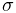. Raw weights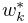are then computed according to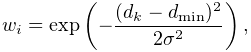and then normalized to form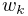.

The method

void setInverseSquareWeighting()

reverts the weighting function back to inverse-square weighting.

It is also possible to specify a custom weighting function by implementing a subclass of SkinWeightingFunction. Subclasses must implement the function

void computeWeights (
double[] weights, Point3d pos, NearestPoint[] nearestPnts);

in which the weights for each master body are computed and returned in weights. pos gives the initial position of the vertex (or attached point) being skinning, while nearestPnts provides information about the distance from pos to each of the master bodies, using an array of SkinMeshBody.NearestPoint objects:

class NearestPoint {
public Point3d nearPoint;   // nearest point on the body
public double distance;     // distance to the body
public ModelComponent body; // master body (either Frame or FemModel3d)
}

Once an instance of SkinWeightingFunction has been created, it can be set as the skin mesh weighting function by calling

void setWeightingFunction (SkinWeightingFunction fxn)

Subsequent calls to computeAllVertexConnections(), or the addMarker or computeAttachment methods described in Section 9.4, will then employ the specified weighting.

As an example, imagine an application wishes to compute weights according to an inverse-cubic weighting function, such that to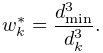A subclass of SkinWeightingFunction implementing this could then be defined as

class MyWeighting extends SkinWeightingFunction {
// implements inverse-cubic weighting
public void computeWeights (
double[] weights, Point3d pos, NearestPoint[] nearestPnts) {
// find minimum distance to all the master bodies
double dmin = Double.POSITIVE_INFINITY;
for (int i=0; i<nearestPnts.length; i++) {
if (nearestPnts[i].distance < dmin) {
dmin = nearestPnts[i].distance;
}
}
double sumw = 0; // sum of all weights (for normalizing)
// compute raw weights:
for (int i=0; i<nearestPnts.length; i++) {
double d = nearestPnts[i].distance;
double w;
if (d == dmin) {
w = 1;  // handles case where dmin = d = 0
}
else {
w = dmin*dmin*dmin/(d*d*d);
}
weights[i] = w;
sumw += w;
}
// normalize the weights:
for (int i=0; i<nearestPnts.length; i++) {
weights[i] /= sumw;
}
}
}

and then set as the weighting function using the code fragment:

SkinMeshBody skinMesh;
// ...
skinMesh.setWeightingFunction (new MyWeighting());

The current weighting function for a skin mesh can be queried using

SkinWeightingFunction getWeightingFunction()

The inverse-square and Gaussian weighting methods described above are implemented using the system-provided SkinWeightingFunction subclasses InverseSquareWeighting and GaussianWeighting, respectively.

## 9.3.1 Setting weights explicitly

As an alternative to the weighting function, applications can also create connections to vertices or points in which the weights are explicitly specified. This allows for situations in which a weighting function is unable to properly specify all the weights correctly.

When a mesh is initially added to a skin body, via either the constructor SkinMeshBody(mesh), or by a call to setMesh(mesh), all master body connections are cleared and the vertex position is “fixed” to its initial position, also known as its base position. After the master bodies have been added, vertex connections can be created by calling computeAllVertexConnections(), as described above. However, connections can also be created on a per-vertex basis, using the method

void computeVertexConnections (int vidx, VectorNd weights)

where vidx is the index of the desired vertex and weights is an optional argument which if non-null explicitly specifies the connection weights. A sketch example of how this can be used is given in the following code fragment:

VectorNd weights = new VectorNd (skinMesh.numMasterBodies());
// compute connections for each vertex
for (int i=0; i<skinMesh.numVertices(); i++) {
// ... compute connections weights as required ...
skinMesh.computeVertexConnections (i, weights);
}

For comparison, it should be noted that the code fragment

for (int i=0; i<skinMesh.numVertices(); i++) {
skinMesh.computeVertexConnections (i, null);
}

in which weights are not explicitly specified, is is equivalent to calling computeAllVertexConnections().

If necessary, after vertex connections have been computed, they can also be cleared, using the method

void clearVertexConnections (int vidx)

This will disconnect the vertex with index vidx from the master bodies, and set its base weighting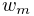(equation 9.1) to 1, so that it will remain fixed to its initial position.

In some special cases, it may be desirable for an application to set attachment base weights to some value other than 0 when connections are present. Base weights for vertex attachments can be queried and set using the methods

double getVertexBaseWeight (int vidx)
void setVertexBaseWeight (int vidx, double weight, boolean normalize)

In the second method, the argument normalize, if true, causes the weights of the other connections to be scaled so that the total weight sum remains the same. For skin markers and point attachments (Section 9.4), base weights can be set by calling the equivalent PointSkinAttachment methods

double getBaseWeight ()
void setBaseWeight (double weight, boolean normalize)

(If needed, the attachment for a skin marker can be obtained by calling its getAttachment() method.) In addition, base weights can also be specified in the weights argument to the method computeVertexConnections(vidx,weights), as well as the methods addMarker(name,pos,weights) and createPointAttachment(pnt,weights) described in Section 9.4. This is done by giving weights a size equal to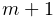, where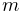is the number of master bodies, and specifying the base weight in the last location.